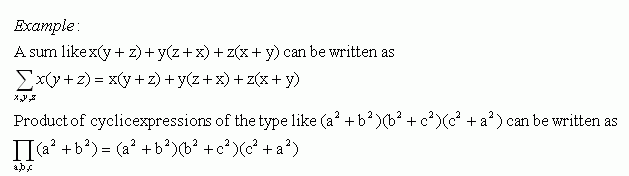Email us to get an instant 20% discount on highly effective K-12 Math & English kwizNET Programs!

#### Online Quiz (WorksheetABCD)

Questions Per Quiz = 2 4 6 8 10

### High School Mathematics6.19 Symmetric and Cyclic Expressions

Symmetric:
An expression f(x,y) is said to be symmetric if f(x,y) = f(y,x).

Example:
f(x,y) = ax + b + ay
If we interchange x and y, we can get the expression ay + b + ax which is same as ax + b + ay.

The sum, difference, product and quotient of two symmetric expressions are again symmetric

Cyclic Expressions:
An expression f(x,y,z) is said to be a cyclic expression if f(x,y,z) = f(y,z,x).
For the sake of brevity we use the symbols ĺ and Ő to write the cyclic expressions.
ĺ is used for sum of terms and Ő is used for product of terms.Directions: Choose the correct answer. Also write at least 5 examples of your own.
 Q 1: x2 + y2 + z2, is this equation symmetry or not?YesNo Q 2: Expand, ĺ a2b. (Variables 'a' and 'b')b2aa2ba2b + b2aa2b + ba Question 3: This question is available to subscribers only! Question 4: This question is available to subscribers only!

#### Subscription to kwizNET Learning System offers the following benefits:

• Unrestricted access to grade appropriate lessons, quizzes, & printable worksheets
• Instant scoring of online quizzes
• Progress tracking and award certificates to keep your student motivated
• Unlimited practice with auto-generated 'WIZ MATH' quizzes